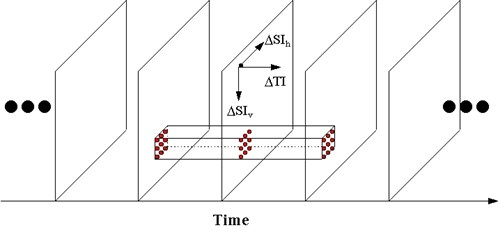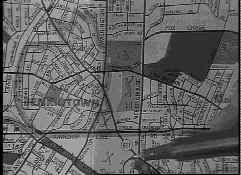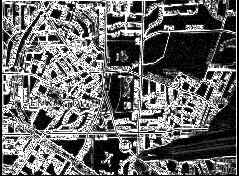Institute for Telecommunication Sciences / Research Topics / Video Quality Research / Guides and Tutorials / ANSI Video Quality Tutorial / Gradient Model of Video Quality

# Gradient Model of Video QualityIntroduction

The gradient model of video quality uses gradients, or slopes, of the input and output images to derive objective video quality metrics. These gradients represent instantaneous changes in the pixel value over time and space. The three types of gradients that have proven useful are depicted in the figure below. These are the spatial information in the horizontal direction (SIh ), the spatial information in the vertical direction (SIv), and the temporal information (TI). Video quality metrics based on spatial and temporal gradients have produced coefficients of correlations to subjective mean opinion score (i.e., where a panel of viewers rate the perceived quality of the video picture) from 0.85 to 0.95. These excellent correlations hold for a wide range of analog and digital video systems and test scenes.

Compression of Quality Information

It is possible to obtain excellent metrics of video quality without comparing each pixel in the input and output images. Rather, summary statistics, or features, can first be extracted from processed versions of the input and output images, and these summary statistics can be compared to produce a parameter or metric. Summary statistics can be computed over simple spatial-temporal sub-regions such as the one shown by the boxed area in the above figure.

Significantly, parameters based on scalar features (i.e., a single quantity of information per video frame) have produced good correlations to subjective quality. This demonstrates that the amount of reference information that is required from the video input to perform meaningful quality measurements is much less than the entire video frame. This important new idea of compressing the reference information for performing video quality measurements has significant advantages, particularly for such applications as long-term maintenance and monitoring of network performance, fault detection, automatic quality monitoring, and dynamic optimization of limited network resources. Since the extracted video quality features require very few bits to transmit, it is possible to perform in-service end-to-end performance monitoring. A historical record of the output scalar features requires very little storage, so they may be efficiently archived for future reference. Then, changes in the digital video system over time can be detected by simply comparing these past historical records with current output feature values.

Example Scalar Features for Video Quality Metrics

The first example to be presented is scalar features based on statistics of spatial gradients in the vicinity of image pixels. These spatial statistics are indicators of the amount and type of spatial information, or edges, in the video scene. The second example is scalar features based on the statistics of temporal changes to the image pixels. These temporal statistics are indicators of the amount and type of temporal information, or motion, in the video scene from one frame to the next. Spatial and temporal gradients are useful because they produce measures of the amount of perceptual information, or change in the video scene.

Spatial Information (SI) Features

The figure below demonstrates the process used to extract a spatial information (SI) feature from a sampled video frame. Gradient or edge enhancement algorithms (i.e., Sobel filters) are applied to the video frame. At each image pixel, two gradient operators are applied to enhance both vertical differences (i.e., horizontal edges) and horizontal differences (i.e., vertical edges). Thus, at each image pixel, one can obtain estimates of the magnitude and direction of the spatial gradient (the right-hand image in the figure below shows magnitude only, called SIr in ANSI T1.801.03-1996). A statistic is then calculated on a selected subregion of the spatial gradient image to produce a scalar quantity. Examples of useful scalar features that can be computed from spatial gradient images include total root mean square energy (this spatial information feature is denoted as SIrms in ANSI T1.801.03), and total energy that is of magnitude greater than rmin and within D q radians of the horizontal and vertical directions (denoted as HV(D q , rmin) in ANSI T1.801.03). Parameters for detecting and quantifying digital video impairments such as blurring, tiling, and edge busyness are measured using time histories of SI features.EdgeEnhancement=>Image SIr of Image

Temporal Information (TI) Features

The figure below demonstrates the process used to extract temporal information (TI) features from a video frame sampled at time n (i.e., frame n in the figure). First, temporal gradients are calculated for each image pixel by subtracting, pixel by pixel, frame n-1 (i.e., one frame earlier in time) from frame n. The right-most image shows the absolute magnitude of the temporal gradient and, in this case, the larger temporal gradients (white areas) are due to subject motion. A statistical process, calculated on a selected subregion of the temporal gradient image, is used to produce a scalar feature. An example of a useful scalar feature that can be computed from temporal gradient images is the total root mean square energy (this temporal information feature is denoted as TIrms in ANSI T1.801.03). Parameters for detecting and quantifying digital video impairments such as jerkiness, quantization noise, and error blocks are measured using time histories of temporal information features.-=Frame n Frame n-1 TI